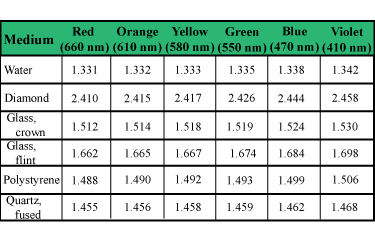# Problem: Consider a diamond surrounded by air.A) By how much (in degrees) do the critical angles for total internal reflection differ for red (660 nm) light compared to violet (410 nm) light?

###### FREE Expert Solution

Critica angle:

$\overline{){{\mathbf{\theta }}}_{{\mathbf{c}}}{\mathbf{=}}{\mathbf{s}}{\mathbf{i}}{{\mathbf{n}}}^{\mathbf{-}\mathbf{1}}{\mathbf{\left(}}\frac{\mathbf{\eta }\mathbf{2}}{\mathbf{\eta }\mathbf{1}}{\mathbf{\right)}}}$

η2 = ηair = 1

η1 = ηincident

θc,red - θc,violet = sin-1(1/ηred) - sin-1(1/ηviolet###### Problem DetailsConsider a diamond surrounded by air.

A) By how much (in degrees) do the critical angles for total internal reflection differ for red (660 nm) light compared to violet (410 nm) light?